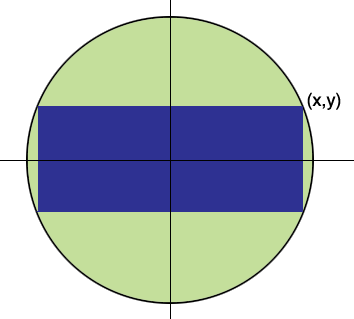SEARCH HOMEMath Central Quandaries & QueriesQuestion from sadiq, a student: here is the question, in my mathematics book there is equation of the area of the rectangle inscribed in a circle having equation x^2+y^2=a^2 and the area of rectangle is 4xy=4x(a^2-b^2)^1/2 i don't know what is b but a is surely the radius (i want the derivation for the area of rectangle).Hi,If (x, y) is a vertex of the rectangle then the rectangle is 2x units by 2y units and hence its area is 4xy square units. Since (x, y) is on the circle you also know that x2 + y2 = a2 and hence y2 = a2 - x2 or y = (a2 - x2)1/2. Substituting this value for y into the expression for the area of the rectangle gives

4xy = 4x(a2 - x2)1/2.

I think there is a typo in your text book.

HarleyMath Central is supported by the University of Regina and The Pacific Institute for the Mathematical Sciences.